Question
The stoichiometric relationship between the reactants and products of an aqueous reaction can be used to determine different kinds of information about a reaction, such as the volume of a given molarity reactant required to produce a certain amount of product.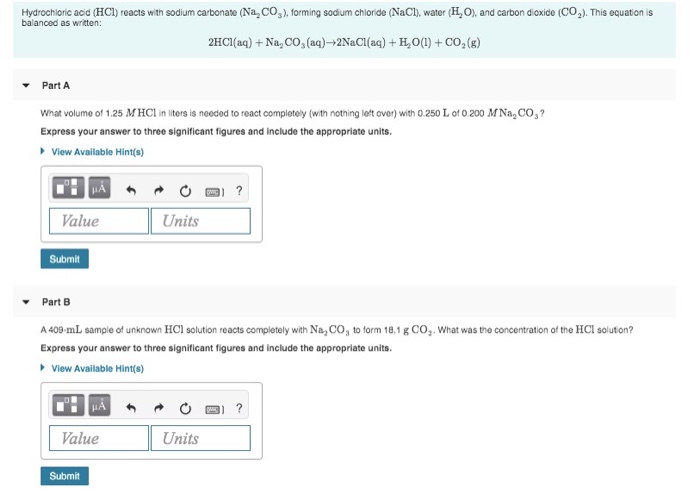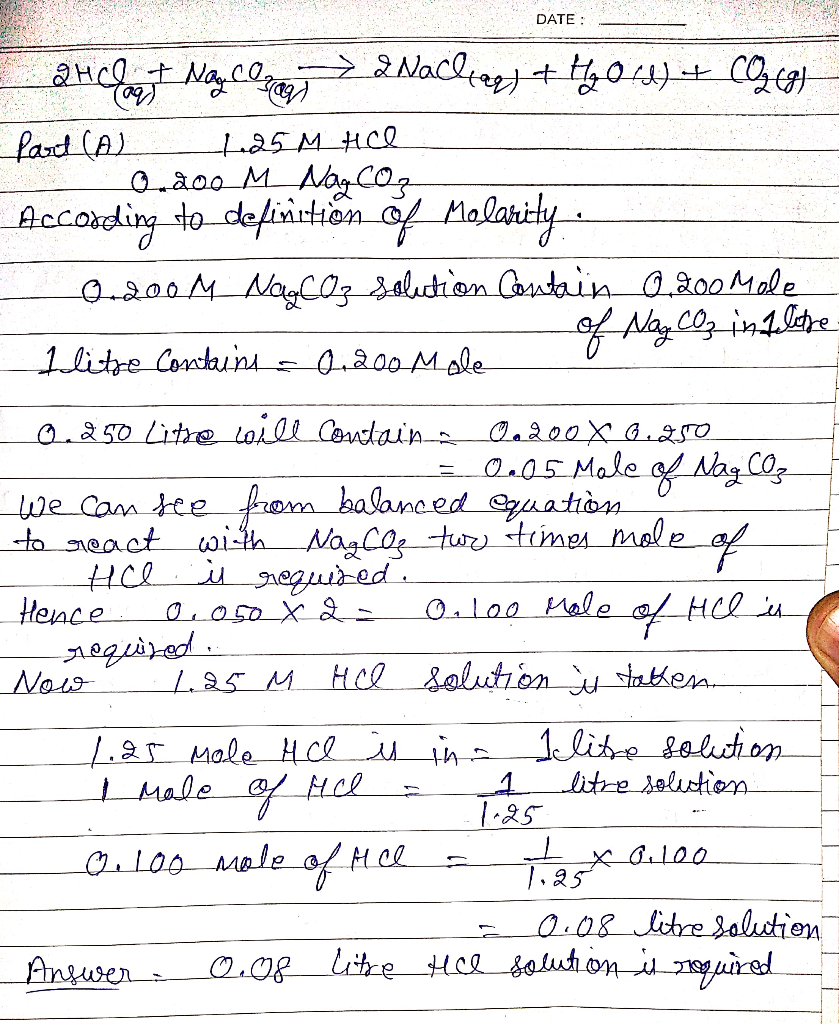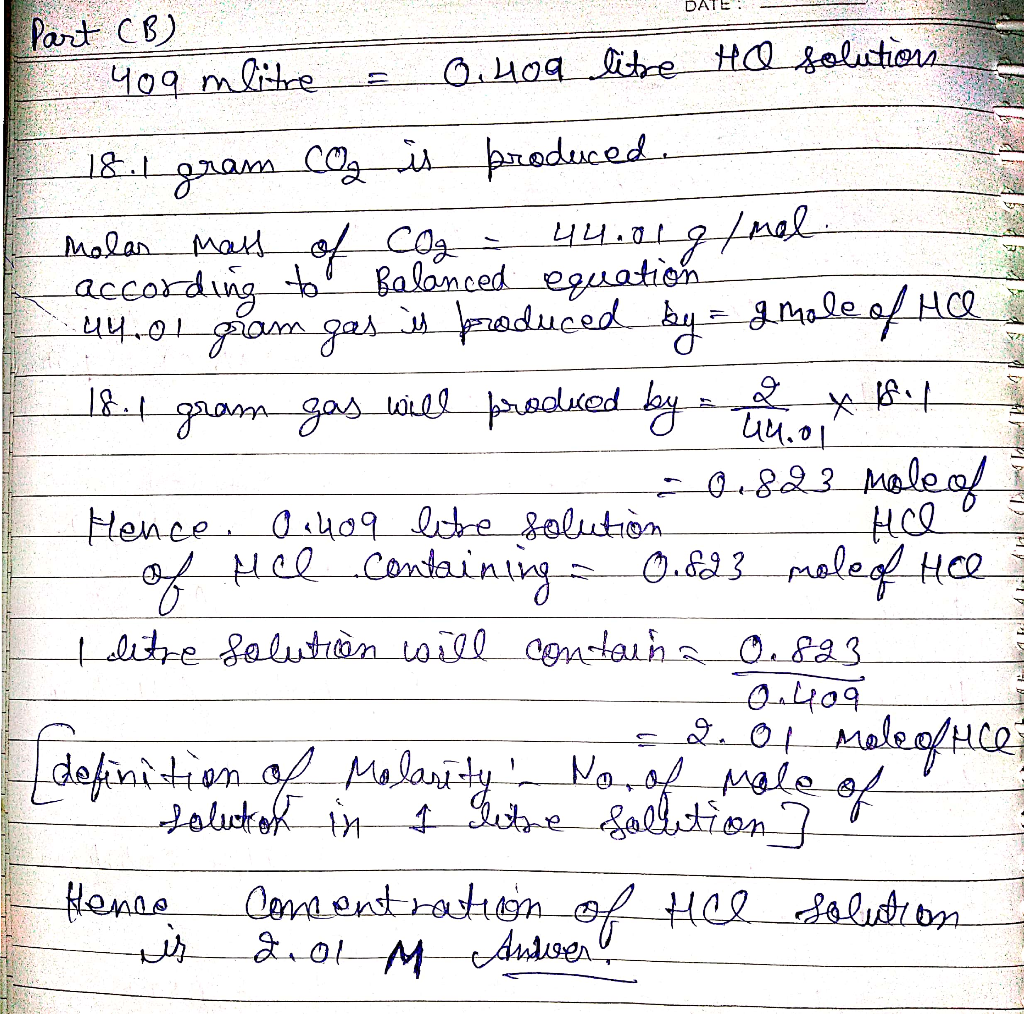#### Earn Coins

Coins can be redeemed for fabulous gifts.

Similar Homework Help Questions
• ### The stoichiometric relationship between the reactants and products of an aqueous reaction can be used to...

The stoichiometric relationship between the reactants and products of an aqueous reaction can be used to determine different kinds of information about a reaction, such as the volume of a given molarity reactant required to produce a certain amount of product. Hydrochloric acid (HCl) reacts with sodium carbonate (Na2CO3), forming sodium chloride (NaCl), water (H2O), and carbon dioxide (CO2). This equation is balanced as written: 2HCl(aq)+Na2CO3(aq)→2NaCl(aq)+H2O(l)+CO2(g) a. What volume of 2.50 M HCl in liters is needed to react completely...

• ### 4. The stoichiometric relationship between the reactants and products of an aqueous reaction can be used...

4. The stoichiometric relationship between the reactants and products of an aqueous reaction can be used to determine different kinds of information about a reaction, such as the volume of a given molarity reactant required to produce a certain amount of product. Hydrochloric acid (HCl) reacts with sodium carbonate (Na2CO3), forming sodium chloride (NaCl), water (H2O), and carbon dioxide (CO2). This equation is balanced as written: 2HCl(aq)+Na2CO3(aq)→2NaCl(aq)+H2O(l)+CO2(g) A.) What volume of 3.00 M HCl in liters is needed to react...

• ### Part A What volume of 1.00 M HCl in liters is needed to react completely (with...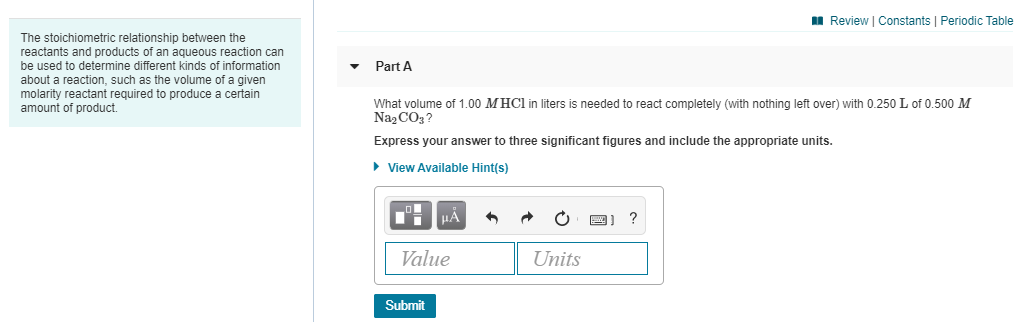Part A What volume of 1.00 M HCl in liters is needed to react completely (with nothing left over) with 0.250 L of 0.500 M Na2CO3? Express your answer to three significant figures and include the appropriate units. Part B A 419-mL sample of unknown HCl solution reacts completely with Na2CO3 to form 12.1 g CO2. What was the concentration of the HCl solution? Express your answer to three significant figures and include the appropriate units. A Review Constants Periodic...

• ### 1. What volume of 1.00 M HCl in liters is needed to react completely (with nothing...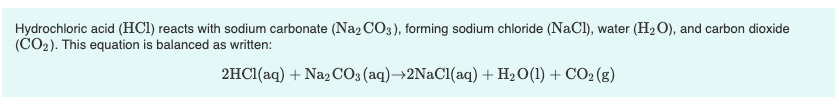1. What volume of 1.00 M HCl in liters is needed to react completely (with nothing left over) with 0.750 L of 0.200 M Na2CO3? Express your answer to three significant figures and include the appropriate units. 2. A 639-mL sample of unknown HCl solution reacts completely with Na2CO3 to form 15.1 g CO2. What was the concentration of the HCl solution? Express your answer to three significant figures and include the appropriate units. Hydrochloric acid (HCl) reacts with sodium...

• ### 9 of 28 A Review Constants | Periodic Table 2HCl(aq) + Na2CO3(aq) +2NaCl(aq) + H2O(l) +...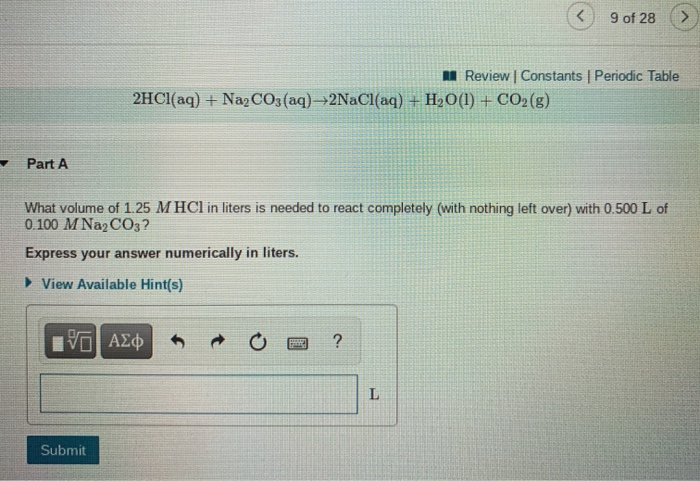9 of 28 A Review Constants | Periodic Table 2HCl(aq) + Na2CO3(aq) +2NaCl(aq) + H2O(l) + CO2(g) Part A What volume of 1.25 M HCl in liters is needed to react completely (with nothing left over) with 0.500 L of 0.100 M Na2CO3? Express your answer numerically in liters. ► View Available Hint(s) ΟΙ ΑΣΦ ? L Submit 9 of 28 Review | Constants Periodic Table Part B A 381-mL sample of unknown HCl solution reacts completely with Na2CO3 to...

• ### Calculate the mass of water produced when 7.44 g of butane reacts with excess oxygen. Express...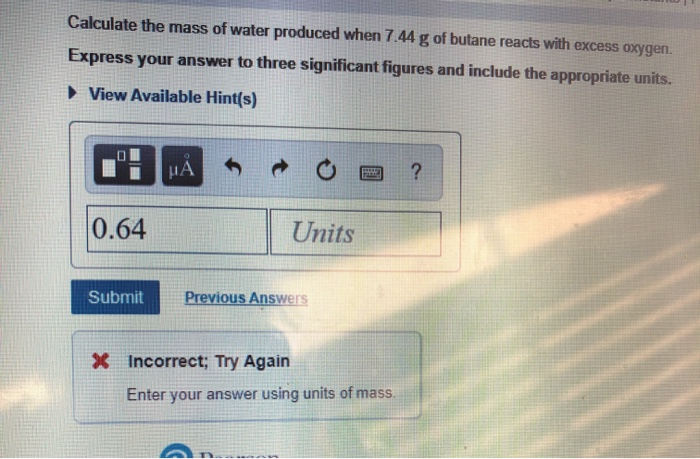Calculate the mass of water produced when 7.44 g of butane reacts with excess oxygen. Express your answer to three significant figures and include the appropriate units. View Available Hint(s) 0.64 Units Submit Previous Answers * Incorrect; Try Again Enter your answer using units of mass. Part Review Constants Periodic Table Calculate the mass of butane needed to produce 61.3 g of carbon dioxide. Express your answer to three significant figures and include the appropriate units. ► View Available Hint(s)...

• ### Part B A 457-mL sample of unknown HCl solution reacts completely with Na,COto form 11.1 g...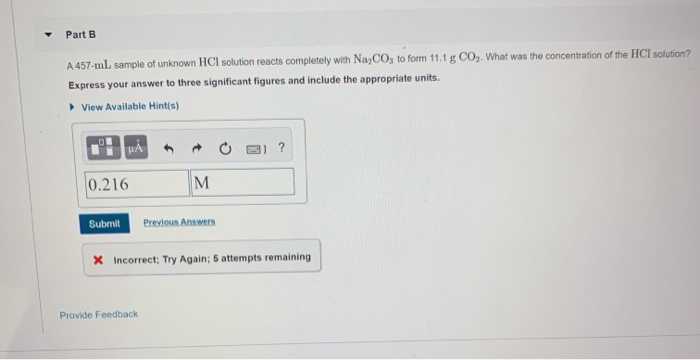Part B A 457-mL sample of unknown HCl solution reacts completely with Na,COto form 11.1 g CO,. What was the concentration of the HCI solution? Express your answer to three significant figures and include the appropriate units View Available Hints) ? .! HAO 110.216 M Submit Previous Answers X Incorrect; Try Again; 5 attempts remaining Provide Feedback

• ### Calcium carbonate (CaCO3) reacts with stomach acid (HCl, hydrochloric acid) according to the following equation: CaCO3(s)...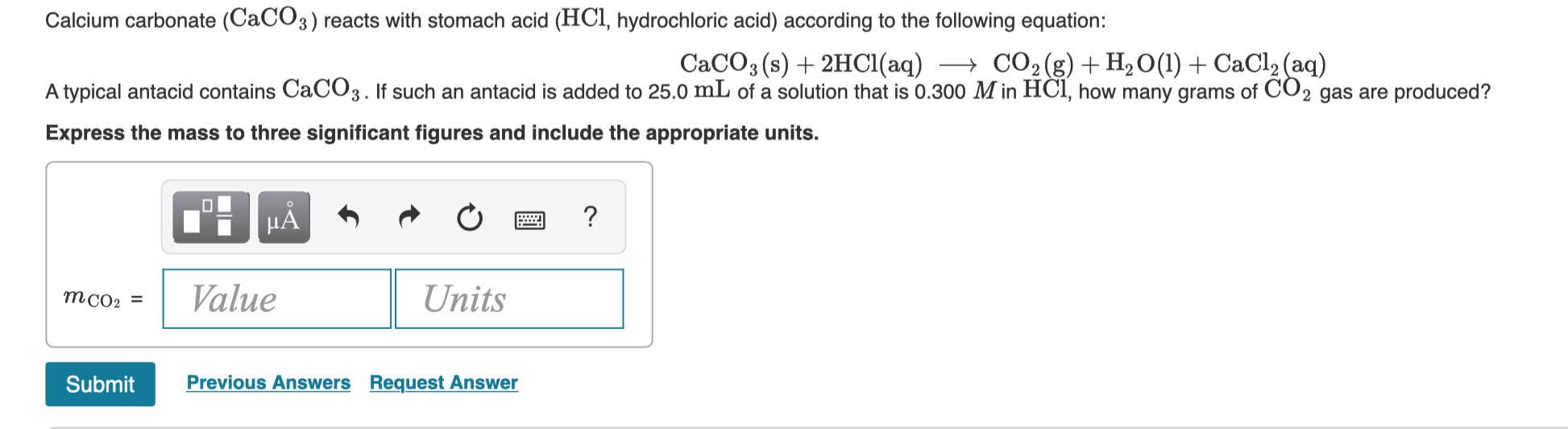Calcium carbonate (CaCO3) reacts with stomach acid (HCl, hydrochloric acid) according to the following equation: CaCO3(s) + 2HCl(aq) + CO2(g) + H2O(l) + CaCl2(aq) A typical antacid contains CaCO3. If such an antacid is added to 25.0 mL of a solution that is 0.300 M in HCl, how many grams of CO2 gas are produced? Express the mass to three significant figures and include the appropriate units. TI MÃ + + + a ? Value Units MCO: = Submit Previous...

• ### Part A What volume of 1.75 M HCl in liters is needed to react completely (with...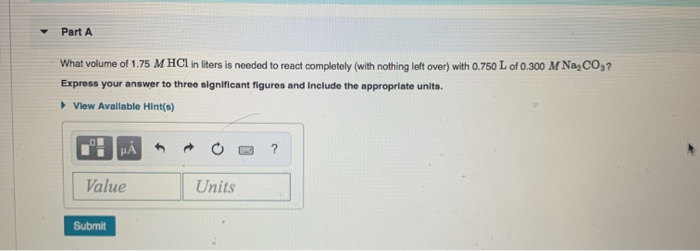Part A What volume of 1.75 M HCl in liters is needed to react completely (with nothing left over) with 0.750 L of 0.300 M NaCO3? Express your answer to three significant figures and include the appropriate units. View Available Hint(s) I HẢ * * 0 -3 ? Value Units Submit

• ### Consider the reaction Mg(s)+Fe2+(aq)→Mg2+(aq)+Fe(s) at 69 ∘C , where [Fe2+]= 3.40 M and [Mg2+]= 0.310 M...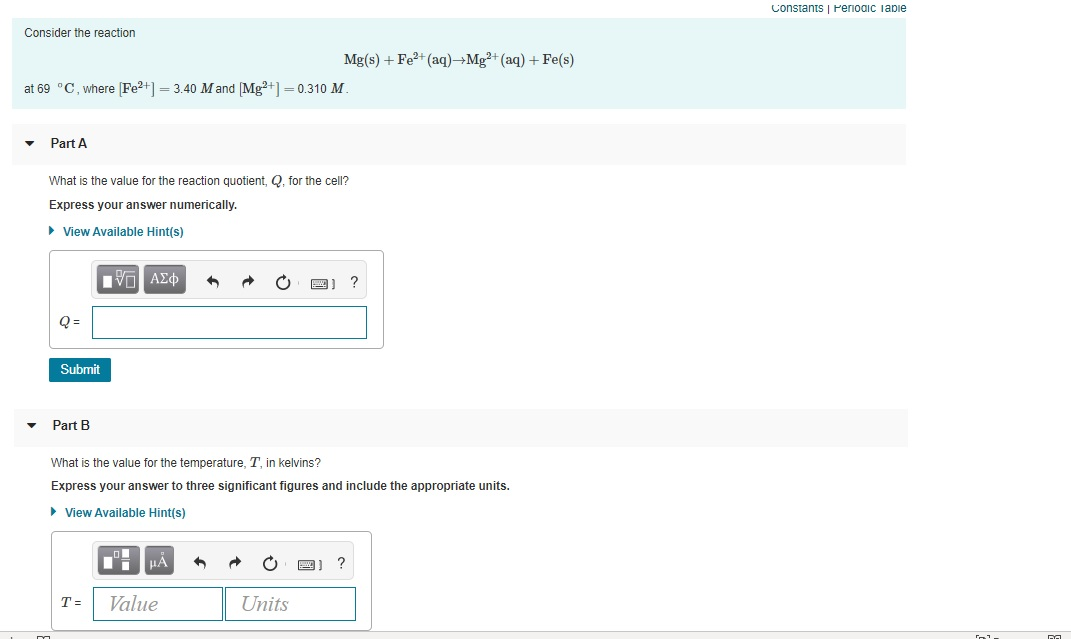Consider the reaction Mg(s)+Fe2+(aq)→Mg2+(aq)+Fe(s) at 69 ∘C , where [Fe2+]= 3.40 M and [Mg2+]= 0.310 M . Constants Periodic Table Consider the reaction Mg(s) + Fe2+ (aq)+Mg2+ (aq) + Fe(s) at 69 °C, where Fe2+1 = 3.40 M and Mg²+] = 0.310 M. Part A What is the value for the reaction quotient, Q. for the cell? Express your answer numerically. ► View Available Hint(s) VO AEQ o oi ? Q= Submit Part B What is the value for the...